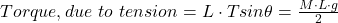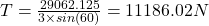## A steel beam of mass 1975 kg and length 3 m is attached to the wall with a pin that can rotate freely on its right side. A cable attached to

Question

A steel beam of mass 1975 kg and length 3 m is attached to the wall with a pin that can rotate freely on its right side. A cable attached to the left end pulls at an angle of 60 degrees above the horizontal and holds the beam horizontal (perpendicular to the wall) in equilibrium.

a) What is the torque due to gravity about an axis containing the pin holding the beam to the wall?

b) What is the torque about the pinned end due to the contact forces between the pin and the beam?

c) In terms of the magnitude of the tension force, T, and any relevant angles, find a symbolic expression for the torque due to the tension in the cable about the axis containing the pin (make sure to include the correct sign). Your answer may contain the symbols M, L, T, g, and mathematical functions.

d) Keeping in mind that the system is in equilibrium, find the magnitude of the tension force.

in progress 0
2 months 2021-07-22T10:05:01+00:00 1 Answers 1 views 0

a) 29062.125 N·m

b) 0 N·m

c)d) T = 11186.02 N

Explanation:

We are given

Beam mass = 1975 kg

Beam length = 3 m

Cable angle = 60° above horizontal

a) We have the formula for torque given as follows;

Torque about the pin = Force × Perpendicular distance of force from pin

Where the force = Force due to gravity or weight, we have

Weight = Mass × Acceleration due to gravity = 1975 × 9.81 = 19374.75 N

Point of action of force = Midpoint for a uniform beam = length/2

∴ Point of action of force = 3/2 = 1.5 m

Torque due to gravity = 19374.75 N × 1.5 m = 29062.125 N·m

b) Torque about the pinned end due to the contact forces between the pin and the beam is given by the following relation;

Since the distance from pin to the contact forces between the pin and the beam is 0, the torque which is force multiplied by perpendicular distance is also 0 N·m

c) To find the expression for the tension force, T we find the sum of the moment forces about the pin as follows

Sum of moments about p is given as follows

∑M = 0 gives;

T·sin(θ) × L= M×L/2×g

Therefore torque due to tension is given by the following expressiond) Plugging in the values in the torque due to tension equation, we have;Therefore, we make the tension force, T the subject of the formula hence